## The Trouble with Watts

I recently read an article in a well-known consumer-facing technical hobby magazine, where the author used the phrase, “watts per day.”  This is an erroneous concept (the technically-trained author should have known better), which illustrates a matter that is often confusing to the average person.

“Watts per day” is not a meaningful unit of measurement.

The watt (W) is a unit of power, which is defined as the rate at which energy is transferred or used. One watt is equal to one joule per second (J/s).

The unit of energy is typically measured in joules (J), but it is also commonly measured in watt-hours (Wh) or kilowatt-hours (kWh). One watt-hour is equal to one watt of power used for one hour, and one kilowatt-hour is equal to one kilowatt (1000 watts) of power used for one hour.

In other words, the “watt” conveys the notion of the rate of energy consumption. When we pay for energy consumed, we pay for kilowatt-hours.

Therefore, if you see the phrase “watts per day,” it is likely that the author was trying to convey the notion of power used over a period of one day, and the correct usage would be to express it in terms of watt-hours (or kilowatt-hours) per day.

As an example: if a piece of equipment is rated at 100 watts, and the equipment is used for 8 hours every day, then we can say that the equipment consumes 800 watt-hours of energy (not power) per day.

agc

## What is the Ecliptic? — Sky & Telescope, September 2022 — Errata

The inaugural Sky & Telescope article of Beginner’s Space – What is the Ecliptic? already has a serious error: the Earth is drawn incorrectly! Even in this artful depiction, it’s clear that the orientation of the continents should be rotated clockwise by 23.4°.

In the equatorial-azimuthal projection used there, the Geographic North Pole of the Earth (which of course projects to the North celestial pole) should emanate from above central Canada, not from above eastern Canada, as drawn in the article. Similarly, the Celestial equator (not the Ecliptic) should be drawn as the projection of the Earth’s equator (as correctly described in the article), which of course runs across the top of South America.

Let’s hope not too many new readers are confused!

agc

## Image Stacking — Sky & Telescope, April 2022 — Errata

The following errata correct the April 2022 Sky & Telescope Article, Image Stacking Demystified, by Richard S. Wright, Jr.

1. The cumulative image noise is proportional to the square root of the number of frames being stacked.
2. Mathematically, the shot noise from the imager and associated electronics can be considered to be added to the signal.
3. The horizontal axis in both graphs on p.56 should be labeled “number of stacked frames,” and the vertical axis should be labeled “quantity.”

EXPLANATION

1.  The S&T author writes, “Shot noise is also quantifiable — it’s simply the square root of the signal value.” This is incorrect, as are the numerical examples that follow the statement.1 The noise and signal components are separate entities, and one cannot say that one of them is a function (square root) of the other.  (Actually, some systems do have an interaction between the two, but those are usually 2nd-order, minor effects, and are not relevant in the image stacking situation.)  The noise value is completely determined by the physics of the imaging device and the transistors in the related electronics, and is independent of the signal – it’s even there when there is no signal (e.g., a dark frame).  The incorrect statement would imply that a dark frame has zero noise, which is not true: in addition to fixed pattern noise (which we can reduce by subtracting a dark frame when doing advanced image processing), the dark frame will have its own random noise, too.

What is really happening is the following.  When we stack multiple images, we are literally adding the images together, pixel-by-pixel.  That means that the signal components get added together, and so do the noise components.  When the images are properly aligned (registered), the signal components at each pixel from one frame to the next add together as correlated data, since they are part of the same image. This combination is literally a simple addition, so image stacking increases the signal component in proportion to the number of frames being stacked.

However, the noise component at each pixel from frame to frame is uncorrelated, because it is a random process.  The noise components add together as orthogonal vectors, which means that the noise value increases by the square root of the number of frames being added together.  (The stacked images are then re-scaled, so that the resulting image doesn’t get progressively brighter everywhere – but this, of course, scales the noise by the same amount.)  The signal-to-noise ratio improvement is therefore proportional to the square-root of the number of frames that are stacked.

2.  The S&T author writes, “It’s important to bear in mind that this noise is not something that gets added so much as something that’s missing.” This is incorrect.  The mathematical modelling and analysis of signals with noise accounts for each of these elements as an added component; there is nothing “missing” from the original signal, which still exists in the image capture.  In practice, this can be readily seen by using a spectrum analyzer, which will show that the signal and noise are separate components.  The shot noise from the imager and associated electronics should be considered to be added to the signal.

3.  The graphs on p.56 are labeled incorrectly. The horizontal axis in both graphs should be labeled “number of stacked frames,” and the vertical axis should be labeled “quantity,” as it represents either signal or noise in the left-hand graph, and signal-to-noise ratio in the right-hand graph.

—agc

FOOTNOTE (1):

There is a different quantity, known as photon noise, which is characterized as the square root of the photon signal, but this is not the dominant factor in our calculation of signal-to-noise ratio, because we are considering the net effect over a set of stacked frames.

REFERENCES:

Wikipedia: Shot Noise – note the discussion regarding “square root of the expected number of events.”

Wikipedia: Gaussian Noise – “values at any pair of times are identically distributed and statistically independent (and hence uncorrelated).”

Philippe Cattin, Image Restoration: Introduction to Signal and Image Processing.

Robert Fisher, et al, Image Synthesis — Noise Generation.

## ITU-R PUBLISHES TWO DOCUMENTS on PLANNING and TESTING of ATSC 3.0 SERVICE

#### AGC Systems Key Participant in ITU-R Rapporteur Group and ATSC Planning Teams

The International Telecommunications Union (ITU) has published two new documents that support the planning and testing of ATSC 3.0 systems.  The documents are:

• ITU-R Recommendation BT.2033-2 : Planning criteria, including protection ratios, for second generation of digital terrestrial television broadcasting systems in the VHF/UHF bands.
• ITU-R Report BT.2495 : Methods for laboratory and field measurements for the assessment of ATSC 3.0 reception quality.

This brings the total number of new ATSC-related documents to five, which includes the Handbook on Digital Terrestrial Television Broadcasting networks and systems implementation, which was updated last year.

Several ATSC-3.0-related documents are also in the ITU-R deliberation process, which are expected to result in additional publications. AGC Systems has been involved in this process for several years, and leads several initiatives to develop these documents.

The International Telecommunication Union is the United Nations specialized agency for information and communication technologies (ICTs). Founded in 1865 to facilitate international connectivity in communications networks, ITU allocates global radio spectrum and satellite orbits, develops technical standards that ensure networks and technologies seamlessly interconnect, and strives to improve access to ICTs to underserved communities worldwide.

The Advanced Television Systems Committee, Inc. is an international, non-profit organization developing voluntary standards for digital television.  ATSC member organizations represent the broadcast, broadcast equipment, motion picture, consumer electronics, computer, cable, satellite, and semiconductor industries.  The ATSC mission is to create and foster implementation of voluntary Standards and Recommended Practices to advance terrestrial digital television broadcasting, and to facilitate interoperability with other media.

# # #

## Cugnini to Present Talk on New Audio/Video Technologies

AGC Systems’ President Aldo Cugnini will deliver an online talk, entitled “New Audio/Video/Wireless Technologies For Home Entertainment.”  Scheduled for Thursday, November 18, 2021, 6:30PM EST, and hosted by the IEEE Consultants’ Network of Northern New Jersey, the talk will explain how new technologies like UHDTV, HDR, and HEVC enable audio and video devices to efficiently deliver the latest entertainment to consumers.

The talk is free, and can be accessed by registering here.

## Carrier-to-noise ratio

Eb/N0 is closely related to the carrier-to-noise ratio (CNR or C/N), i.e. the signal-to-noise ratio (SNR) of the received signal, after the receiver filter but before detection:where

fb is the channel data rate (net bitrate), and
B is the channel bandwidth

The equivalent expression in logarithmic form (dB):Source:  Wikipedia

## Modulation 101

Modulation is the process of imparting a signal, usually audio, video, or data, onto a high-frequency carrier, for the purpose of transmitting that signal over a distance.

Let’s take a carrier signal, $cos(ω_c t)$ and a modulating signal, $cos(ω_m t)$, where $ω = 2 \pi f$, and f is the signal frequency.

Amplitude modulation is simply the product of the carrier signal and (1 + modulating signal):

$$\displaystyle AM(t) = cos(ω_c t) \times [1 + cos(ω_m t)] ,$$

which multiplies out as: $AM(t) = cos(ω_c t) + cos(ω_c t)\times cos(ω_m t) ] .$ A carrier modulated by a sine wave is shown in the following example.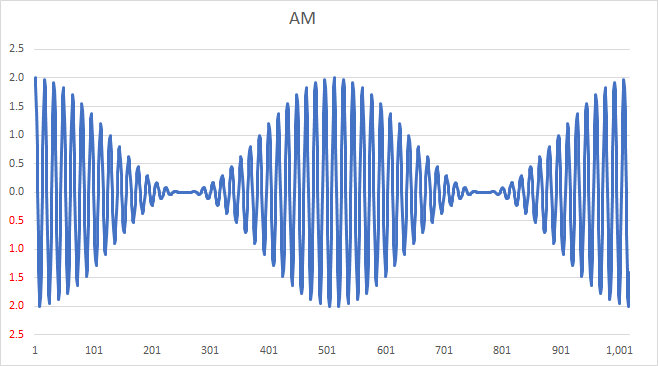Note that such a signal is relatively easy to demodulate: a simple rectifier and low-pass filter will recover the modulation from this signal, as you can visualize by “erasing” the negative portion of the signal and averaging over the remaining waveform.  Such a process is called envelope detection.

To analyze the composition of this signal, we take the trig product identity, $cos(x)\,cos(y) = \frac{1}{2} [ cos(x-y)+cos(x+y) ],$ and apply it to the product term in AM(t), producing the following:

$$\displaystyle AM(t) = cos(ω_c t) + \frac{1}{2} cos(ω_c t - ω_m t) + \frac{1}{2} cos(ω_c t + ω_m t) .$$

From this, we observe an important aspect of the process:  amplitude modulation results in a signal composed of the following three components:

1. the carrier signal, $cos(ω_c t),$
2. a lower sideband signal, $\frac{1}{2} cos(ω_c t - ω_m t),$
3. and an upper sideband signal, $\frac{1}{2} cos(ω_c t + ω_m t).$

By the way, the reason for the “1 + ” term in the modulation equation above is that it specifically generates the carrier component in the modulated signal. Without it, we would have the following Double-Sideband-Suppressed Carrier signal, which should make it apparent that we can’t use a simple envelope detector to demodulate; note how the envelope “crosses over” itself:An analysis of modulation is aided by using a more complex modulating signal.  A ramp signal is comprised of a fundamental sinusoid and integer harmonics of that fundamental.  For illustration purposes, we will take an approximation that uses the fundamental and the next 8 harmonics. This modulating signal is shown below, as a function of time.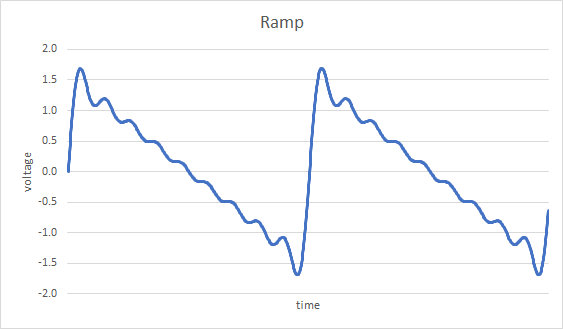The spectrum of this signal, i.e., a plot of the frequency components versus level, is shown next; it consists of a fundamental (at “1”), followed by a series of harmonics with decreasing levels.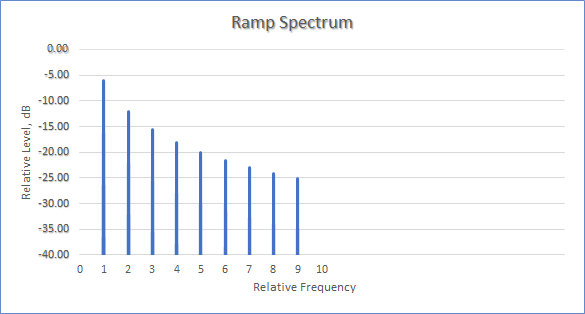If we amplitude modulate a carrier with this ramp signal, we get the following time-varying signal; note again that this signal can be demodulated by an envelope detector: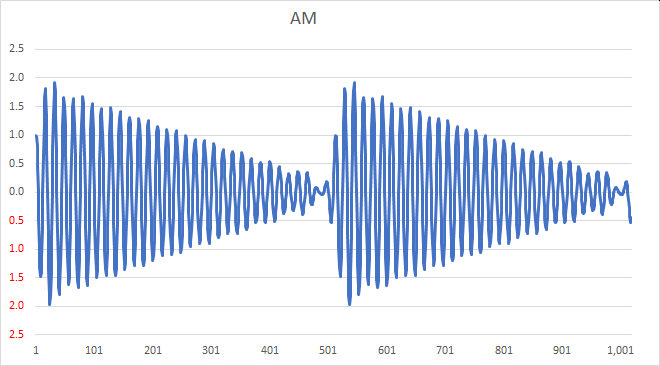The spectrum of the modulated ramp signal follows; note that there is a carrier at “0” and sidebands extending in both the positive and negative frequency directions. (In practice, this zero point would actually be at some high frequency, such as at 7 MHz for example. The spacing of the individual components in this example would be exactly that of the frequency of the fundamental component of the ramp signal.)Recall from our earlier discussion that amplitude modulation results in a signal composed of the three components, the carrier signal, a lower sideband signal, and an upper sideband signal. Note the following as well: because the lower sideband component has a negative modulating-frequency term ($cos(ω_c t - ω_m t),$ for a sine wave) the spectrum of the lower sideband is reversed compared with that of the upper sideband (and that of the baseband modulating signal).

We can also see from this example that amplitude modulation is rather wasteful of spectrum space, if our goal is to take up as little bandwidth as possible.  For one, the two sidebands are merely reflections of each other, i.e., each one carries the same information content.  For another, the carrier itself is unnecessary for the communication of the modulating signal as well — something that wastes power on the transmission side.

Taking that into account, we can choose to transmit only one sideband, resulting in a Single Sideband (SSB) Transmission. If we transmit only the lower sideband, its spectrum will look like this (note that the carrier is also absent):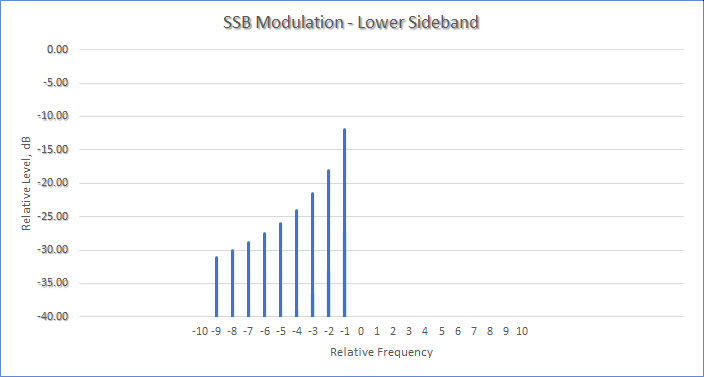SSB modulation can be implemented using a variety of methods, including an analog filter, or phase-shift network (PSN) quadrature modulation.  (For a clue as to how PSN works, look up and calculate the result of adding $cos(x) cos(y) + sin(x) sin(y)$.)

The challenge in receiving this signal is how to demodulate it, as we can see from its time-domain plot: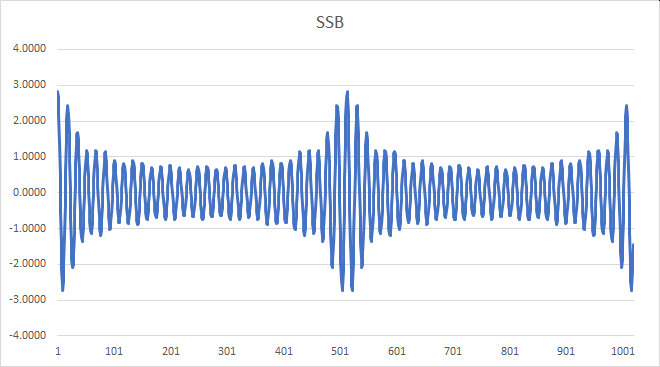As compared with amplitude modulation, a SSB signal cannot be demodulated with an envelope detector, because the envelope is no longer a faithful representation of the original signal.  One way to demodulate it is to frequency-shift the signal down to its original range of baseband frequencies, by using a product detector which mixes it with the output of a beat frequency oscillator (BFO).

One can appreciate that, if the demodulator BFO is not exactly at the original carrier frequency, the resulting demodulated signal will be frequency-shifted up or down by the amount of the error, resulting in a kind of “Donald Duck”-sounding voice signal.  While this was often an issue with analog transmitters and receivers, whose carrier frequencies were imprecise, and would drift over time, modern digital equipment is so accurate that a near-perfect re-synchronization is not difficult to achieve.

— agc

/ / /

Here’s a brief summary on how to make a good recording:1. Don’t use the video capture app in your device, because we won’t be using any video, as it complicates the production.
2. Download an app to record your instrumental part, and learn how to use it. Some recommendations:
3. Learn the basic recording features of the app.  Please use the following settings:
• mp3 file type, which could involve a Conversion  step, as offered within the app;
• 44kHz sample rate;
• mono;
• 128kbps bit rate.
4. A separate microphone on a stand will greatly improve the sound. You’ll need an appropriate adapter to connect the mic to your phone or PC.
5.Microphone (or phone) placement will greatly affect the quality of the recording.  Here is a good non-technical article on How to Mic Woodwinds and Brass.
6. To get the best tempo synchronization, you’ll need to listen to a reference recording we’ll send you, while recording your part.  That means you’ll need two devices: one to play back the reference recording (using headphones), and one to record your part.  (There are ways to do this with just one device, but that’s a rather advanced technique that should be left to the more tech-savvy.)
7. If any part of this is too complicated, let us know, and we’ll either solve the issue, or figure out a simpler (but lesser) alternative. Because of the inherent limitations of the process, the better we make each step, the better the overall result will be.
8. Don’t forget to have fun!## Revisiting the Smith Chart

#### Introduction

The advent of low-cost antenna analyzers and vector network analyzers (VNAs) has resulted in a renewed interest in an 80-year old tool called the Smith chart, a graphical device used to view the characteristics of RF transmission lines, antennas, and matching circuits, and to aid in the design of those systems.  But although questions regarding the chart appear in the Amateur Extra License exam, the current Question Pool has a mere 11 questions on the topic, leaving a full appreciation of the tool to the more inquisitive student. Here then, is a brief introduction to the Smith chart.

#### Motivation

Why use a seemingly-archaic tool such as the Smith chart, when calculators and software are readily available?  For one, the chart remains useful as a visualization tool, representing a wealth of information in a very precise and intuitive manner.  In addition, the knowledge gained in its use adds to our general knowledge of radio systems. And that’s what ham radio’s all about, right? Otherwise, we may as well use a telephone or the Internet to communicate over large distances!  And don’t worry; we’ll keep the equations to a minimum (or in footnotes).

Phillip Smith, 1ANB (SK), was an electrical engineer who graduated from Tufts College in 1928, and then went to work in the radio research department at Bell Telephone Laboratories.  Fascinated by the repetitive nature of the impedance variation along a transmission line and its relation to the standing-wave amplitude ratio and wave position, Smith devised an impedance chart in which standing-wave ratios were represented by circles. In 1939, he had an article published in Electronics Magazine describing what later came to be known as the Smith chart.

#### Derivation

The Smith chart provides a concise way to view a number of different characteristics of RF systems, such as impedance and voltage standing-wave ratio (VSWR). We’ll use an example to show how the Smith chart was developed.

Radio operators are inherently familiar with the concept of standing-wave ratio, i.e., a measure of how well a load is matched to a transmission line. A slightly more obscure concept is that of the reflection coefficient. Notated using the Greek letter gamma (Γ), the reflection coefficient describes how much of an electromagnetic wave is reflected by an impedance discontinuity such as a mismatched load. (For a perfect termination, Γ=0, an open termination yields G=1, and a short results in Γ=-1.) Being a complex quantity, Γ can convey both the magnitude and phase of the reflected wave, at a particular frequency., 

We can measure Γ by connecting a VNA to a device under test (DUT), “sweep” a band of interest, and download the data.  Our DUT for this example is a 50-ohm coaxial transmission line terminated by a 40m OCF dipole.

First, let’s plot the real and imaginary components of Γ for this DUT on a rectilinear graph. See Figure 1: the green marker is at 14.175 MHz.

Reading off the chart, we see the following values:

 frequency 14.000 MHz 14.175 MHz 14.350 MHz Γ -0.12 + j 0.26 0.05 + j 0.20 0.08 + j 0.07

Because complex numbers can also be represented in polar form, we can also plot Γ on a polar plot, as in Figure 2. On this plot, the same green marker is now at a magnitude (denoted as |Γ|) of about 0.2 (i.e., its distance from the center or origin), and a phase angle of about 75°. (Note that the curve did not change shape, only the axes of the graph did.) It’s useful to note that the magnitude of the reflection coefficient can never exceed 1.0, as this would imply that power is somehow “created” by the load. This means that Γ is constrained to be within this circular space, such that |Γ|≤ 1.

Smith was no doubt very familiar with the polar type of data presentation, for a practical reason. Back in the day, it would have been rather difficult to measure Γ directly as a complex quantity, due to the limitations of test equipment. But it would have been much easier to measure the polar representation: what was required was an oscilloscope and directional couplers.  With these, one could measure the magnitude and phase angle of a wave reflected from a termination, and plot them on a polar graph of the complex reflection coefficient. A simple trigonometric transformation converts these to the rectangular format.

There are several quantities that can be derived from the reflection coefficient. For one, the VSWR is easily calculated from the magnitude of Γ, and is shown in Figure 3.

Another is the complex impedance, which is also easily calculated from the reflection coefficient at that same “reference plane,” i.e., the point at which the measurement is taken. Because this transformation is so elegant (and important), we present it here:

$$\displaystyle Z_{L}=\frac{1+\Gamma}{1-\Gamma}\cdot Z_{0}$$

Equation 1

 Because RF components behave differently at different frequencies, engineers use complex numbers to describe impedances and related concepts. The impedance measured at the input to an antenna, at a particular frequency, is expressed as a complex number in the form Z = R + jX, where R represents the real value of the impedance (in ohms), X represents the imaginary value (also in ohms), and j is the imaginary constant $\sqrt{-1}$.

 The quantity Γ is also denoted by the scattering parameter s11. S-parameters generally describe the input-output relationship of RF networks; another such s-parameter is s21, which represents the power transferred to a “Port 2” from a “Port 1” of a network.

In other words, knowing the reflection coefficient Γ and the characteristic impedance Z0 of a transmission line connected to a load, we can directly calculate the load impedance ZL.  (As we know, in radio engineering, Z0 is usually 50Ω; video plants commonly use 75Ω, and telephone networks use 600Ω.)

Applying this transformation to our example data, we can produce a graph of the complex load impedance, as in Figure 4, with some interesting observations.  First, although the curve looks similar to that of the reflection coefficient, the shape cannot be the same for the following reason: as we learned before, while the reflection coefficient is bounded to a magnitude of 1, the impedance can take on values from zero to infinity. Indeed, if Γ is close to 1, the load impedance can be very high, usually on the order of thousands of ohms.

The other useful observation is that, while Γ can have both positive and negative real and imaginary components, the real (resistive) part of the complex impedance can only be positive, and can range from 0 to +∞, while the imaginary part can range anywhere from −∞ to +∞.

From a practical standpoint, this means that conventional (linear) representations of both reflection coefficient and impedance suffer from a number of shortcomings: impedance plots that cover a large extent (e.g., traversing a region close to Γ = 1) would be unwieldy and lack precision; simultaneous plots of different systems would be hard (or impossible) to compare; multiple graphs would be needed to convey information that is intrinsically related; and the conventional representations would be limited to some applications of analysis, while impeding the practicality of system design.

 If we denote the phase of Γ by θ, then $\Gamma=\left |\Gamma \right |\cdot (\cos \theta +j\cdot \sin \theta) .$

 $VSWR=\displaystyle \frac{1+\left |\Gamma \right |}{1-\left |\Gamma \right |} .$

 Keep in mind that ZL and Γ are complex quantities, so complex arithmetic must be used!

 There is no such thing as negative resistance (!), except in tunnel diodes, but that’s another story.

#### The Smith chart

Phillip Smith understood these limitations, and set forth to overcome them.  He brilliantly realized that the various concepts of impedance, reflection, and VSWR could become graphically interrelated by means of the right coordinate transformation – and this would solve the “infinite extent” problem, as well.

Smith’s insight was to start with a rectangular grid of complex impedance, and then warp the axes according to the transformation given in Equation 1.  This has the effect of throwing away the negative real (resistance) part, and wrapping the imaginary (reactance) axis so that the points at −∞ and +∞ meet each other. Thus was born his eponymous chart, shown in Figure 5.

The chart consists of a number of internally-tangent circles, and arcs of circles; the number of circles and arcs is simply a function of the desired precision, and charts can also be constructed that “zoom-in” on a particular region of interest. Looking first at the circles, we see they are all internally tangent at a point on the right-hand-side, as seen in Figure 6.  Each of these circles represents a constant real (resistive) component, with the circles ranging from 0 ohms (as indicated at the extreme left of the horizontal line – the real, or resistance, axis) to +∞ ohms (as indicated at the extreme right of the horizontal line).

Intersecting these circles are arcs of circles that have centers lying on a line (not shown) that is perpendicular to the horizontal line, and which all pass through the point at +∞ ohms. (See Figure 7.) Each of these arcs represents a constant imaginary (reactive) component.  The outermost full circle is the imaginary, or reactance, axis.

A few definitions and we’re there. You’ll recall from Equation 1 that the load impedance ZL can be calculated by knowing Γ and the characteristic impedance Z0.  We can rewrite this equation in a form that normalizes the impedances by dividing both sides of the equation by Z0, which produces Equation 2:

$$\displaystyle \frac{Z_{L}}{Z_{0}}=\frac{1+\Gamma}{1-\Gamma}$$

Equation 2

With this normalization, we can use the Smith chart for any characteristic impedance, as long as we remember that the point at the center represents that characteristic impedance. Inspecting the chart, and from the above definitions, we see that the point at the chart center has a value of 1 + 0, i.e., 1.

The last component to observe is the outermost scale, which is calibrated in degrees and fractions of a wavelength.  Among the useful properties of the Smith chart is the fact that adding a length of transmission line has the effect of rotating the complex reflection coefficient clockwise around the chart center, by an angular amount that is proportional to the added electrical length divided by the operating wavelength.

For example, if we add 4 meters of RG-8X transmission line (with velocity factor 0.82) to a system operating at a wavelength of 21.2 meters, the curve will rotate by the following angle:

$$\displaystyle \varphi =\frac{1}{0.82}\cdot \frac{4}{21.2}\cdot 720 \degree = 166 \degree$$

 One wavelength corresponds to two complete rotations around the Smith Chart, hence the 720° factor.  Note that adding multiples of ½ wavelength simply brings us back to the same point!

Returning to our DUT, we can now appreciate the utility of this marvelous tool.  Using the data from Figure 1, if we now take the reflection coefficient curve of our system, and “drop” it onto the Smith chart (see Figure 8), we can read off the following normalized impedance information (and calculate the actual impedance, shown on the table’s third line, by multiplying by Z0 = 50Ω): , 

 14.000 MHz 14.175 MHz 14.350 MHz ZL/Z0 0.68 + j 0.38 1.02 + j 0.41 1.16 + j 0.15 phase(Γ) 116° 76° 38° ZL , Ω 34 + j 19 51 + j 20.5 58 + j 7.5

 It’s important to realize that the scales on the chart are actually performing the calculation for us; we started with a curve of Γ on a conventional polar graph, and copied that same curve onto the Smith chart, where it now represents Z. It’s only the axes that have changed.

 To get the phase of a point, draw line from the center through the point, and extend it to the outer scales, where you can read it off the 0-180° scale.

You may have noticed that the VSWR formula given previously looks curiously similar to the normalized impedance formula given at Equation 2, which leads to another wonderful property of the Smith chart: if you take any point on an impedance plot, and rotate it, around the center, to land on the right-hand half of the resistance axis, the value at that axis is the VSWR at that frequency! (See magnified center at Figure 9.) Note how rotating the green dot would intersect the horizontal axis at the value 1.5, which is what we calculated earlier. In fact, any point on the red circle has a VSWR of that same value. So, when analyzing (or designing) a system with a target VSWR, make sure that the normalized impedance values over the entire frequency of interest remain inside such a circle.

We specifically chose a limited range of frequencies to keep the visuals simple; in practice, extending the frequency over a wider range leads to some very interesting results! Note too, that while many of these measurements were originally done using paper-and-pencil (and drafting tools), we can easily do the equivalent by using graphical software that directly interfaces to a modern VNA.